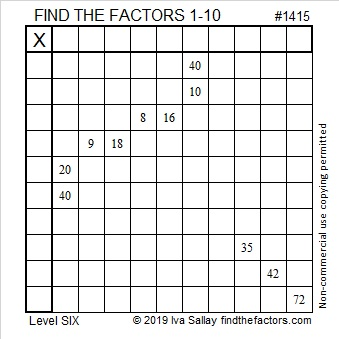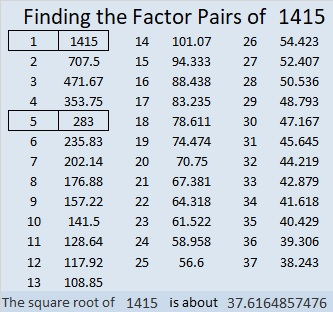# 1415 and Level 6

Very likely when you look at this puzzle common factors of 40 and 10, 8 and 16, 9 and 18, and 20 and 40 will pop into your head. Will they be the right common factors that work with all the other clues in the puzzle to produce a unique solution? Let logic be your guide when finding the factors.Print the puzzles or type the solution in this excel file: 10 Factors 1410-1418

Now I’ll tell you something about the puzzle number, 1415:

• 1415 is a composite number.
• Prime factorization: 1415 = 5 × 283.
• 1415 has no exponents greater than 1 in its prime factorization, so √1415 cannot be simplified.
• The exponents in the prime factorization are 1, and 1. Adding one to each exponent and multiplying we get (1 + 1)(1 + 1) = 2 × 2 = 4. Therefore 1415 has exactly 4 factors.
• The factors of 1415 are outlined with their factor pair partners in the graphic below.1415 is also the hypotenuse of a Pythagorean triple:
849-1132-1415 which is (3-4-5) times 283.